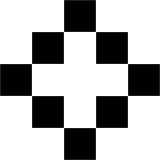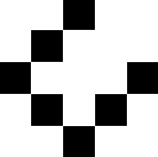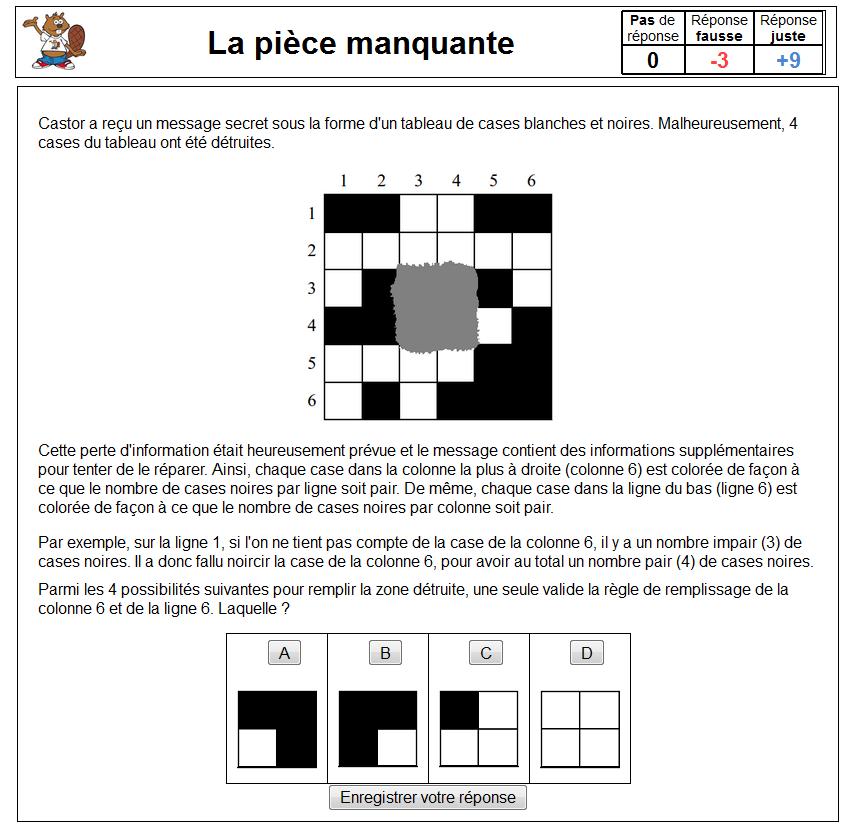﻿ 1, 2, 3, code ! - Cycle 3 activities - Lesson 3.5. (Optional) How to make sure our data are successfully sent | Le site de la Fondation La main à la pâte

# 1, 2, 3, code ! - Cycle 3 activities - Lesson 3.5. (Optional) How to make sure our data are successfully sent

 Summary Students learn that it is possible to detect and correct errors introduced when storing or transferring a file by adding the right information. This lets them do a sort of "magic trick." Key ideas (see Conceptual scenario) "Information" The information is stored in a memory: hard disk or flash memory, for example. Storing and handling data with these memories may introduce errors. There are methods that allow us to detect and correct these errors. This requires increasing the quantity of information to be stored. Inquiry-based methods Experimentation Equipment For each group A pack of 36 cards (standard playing cards or simply cards with one black side and one white side) Glossary Parity bit, data integrity Duration 1 hour

## Foreword

It is possible that students won’t come up with the method known as “parity checking” themselves. We propose two variations of this lesson. In the first (described step by step, below), this method is formulated together as a class. In the second (a quick description at the end of the lesson), the teacher does a “magic trick”, which the students should be able to understand.

## Introductory question

The teacher shows an image similar to that studied at the beginning of Lesson 3.1 and its binary code (here, we have removed the heading of the file “P1 5 5” which indicates that it is a black and white image with 5x5 pixels):0 0 1 0 0 0 1 0 1 0 1 0 0 0 1 0 1 0 1 0 0 0 1 0 0

The teacher asks the students what would happen if, when sending, certain errors occurred, that changed the value of certain pixels. They change any value, and ask a student to come to the board and draw the new image. If the teacher has changed the underlined value, the new image is:0 0 1 0 0 0 1 0 0 0 1 0 0 0 1 0 1 0 1 0 0 0 1 0 0

Teaching note:
This initial question is deliberately chosen to be very easy, which enables the class to recap and refresh what they learned in Lesson 3.1.

## Research (as a class): How to detect an error

The teacher asks the students how we can make sure that the image we receive is indeed the one that was taken. The aim is not yet to correct potential errors, but just to learn how to detect them. Another way of saying it is to ensure the data’s integrity.

Some students may have the idea of adding extra information. They could, for example, calculate the sum of the values line by line and add a number that encodes this value. Or, a simpler (and more practical) option would be to see if the number of 1s on each line is odd or even. In that case, at the end of each line a new number is added so that the number of 1s is always even on every line.

• If the number of 1s on the line is already even, then we add a 0 at the end of the line. The new, completed, line still has an even number of 1s.
• If the number of 1s on the line was uneven, then we would add a 1 at the end of the line. The newly completed line now has an even number of 1s.

When applied to the first image, the result is as follows:0 0 1 0 0 0 1 0 1 0 1 0 0 0 1 0 1 0 1 0 0 0 1 0 0 0 0 1 0 0 1 0 1 0 1 0 0 1 0 0 0 1 0 0 1 0 1 0 0 0 0 1 0 0 1

We added a new column so that, on each line, the number of 1s would be even. The teacher closes their eyes and asks a student to change any value without telling them. All they need to do, then, is count, line by line, the number of 1s to find out which line poses a problem.

Scientific notes

• This method is often used in IT to detect (and correct, see below) errors when copying or sending files.
• The added bit at the end of the line is called a “parity bit”.

## Research (class activity): How to correct the error

The class points out that they can tell there is an error, but they don’t know how to correct it, because we don’t know which pixel has been changed. All we know is the number of the line where there is a problem. In order to find out precisely which value has been changed, we need to know the number of the column.

The teacher asks the class how to do it. This time, it is easier for the students to find the answer. They need to do the same as before, but in columns rather than lines. We count the number of 1s in each column and add another line so that, in each column, the number of 1s is always even.

When applied to the first image, the result is now as follows:0 0 1 0 0 0 1 0 1 0 1 0 0 0 1 0 1 0 1 0 0 0 1 0 0 0 0 1 0 0 1 0 1 0 1 0 0 1 0 0 0 1 0 0 1 0 1 0 0 0 0 1 0 0 1 1 0 0 0 1 0

A student can now change a value anywhere and the teacher shows that they are able to detect this value (by cross-referencing the information in the line and the modified column) and then correcting it.

Scientific note
The parity bit added in the bottom-right corner allows us to detect (and correct) an error that has occurred, not in the bits of the 5x5 grid (the original image) but in the parity bits themselves.

## Exercise (in groups)

Once the method is explained, the teacher divides the class into pairs or groups of four (depending on the equipment available) and hands a set of cards to each pair. The students practice detecting and correcting errors.

• The student being tested covers their eyes while the rest of the group agree on a pattern they make with the cards (each face card is a 1, while each pip card is a 0).
• The student being tested opens their eyes and places the extra cards (“parity bits”).
• The student being tested covers their eyes again while the others turn over 1 card.
• The student being tested should be able, when looking at the parity bits, to figure out which lines and columns were changed, and therefore correct the errors.

Teaching note:

If the class isn’t sure whether the student is using the parity method and not just playing by memory to identify and correct the error, they just need to increase the number of cards (i.e. the number of bits) and avoid creating an easily memorized pattern.

## Group discussion

The teacher makes sure that all students have understood the parity checking method and asks them what would happen if two errors, for example, occur on the same line. The class realizes that, in this case, the error is detectable because the parity of certain columns has changed, but the error cannot be corrected because we don’t know which line has been corrupted.

The class discusses how to minimize this risk. For example, we could increase the number of parity bits (instead of placing one parity bit for every five bits, as we have done here, we can place a parity bit every three bits). The higher the number of parity bits, the easier it is to detect errors. However, on the other hand, the quantity of information to be stored (and later sent) must be increased.

In practice, inserting one parity bit every five bits means increasing the information by 20%, and so the file size also increases by 20%. Depending on the volume of information and the reliability of the storing or sending processes, we can decide if we reduce it to 10% or even 1%: it is a compromise to be made.

## Conclusion and lesson recap activity

The class summarizes together what they learned in this lesson:

• A computer represents all types of information using binary code.
• When saving or handling (copying or sending, for example) data, errors can occur.
• Certain methods, such as parity checking by adding parity bits, allow us to detect and correct these errors. This requires increasing the quantity of information to be stored.

Students write down these conclusions in their science notebook. The teacher updates the "Defining computer science" poster.

## Variation

This lesson can be conducted differently, by breaking away from the inquiry-based method, where the problem posed has no relation to what was studied in previous lessons. However, this variation can be of value, even if it’s just for fun.

• The teacher explains that they will do a “magic trick”.
• The class is divided into four groups. Each group has 25 cards on the table, laid out in a pattern of five lines by five columns.
• The teacher goes from table to table, placing extra cards at the end of each line and column, making sure to keep them even (the number of face cards should be even), without explaining to the students what they are doing.
• They then ask each group to change one card (just one!) and then checks each group, revealing the card that was changed.
• The rest of the lesson can be conducted similarly to what was described above, so that the students discover and grasp the teacher’s method.

The students can do this magic trick at home — success guaranteed!

Note: This variation is inspired by an activity taken from the book Computer Science Unplugged (classic.csunplugged.org ).

## Prolongement

Un exercice en ligne permet de s'essayer à la reconstitution d'une image corrompue.## Extended study for middle school (4th cycle)

The class can extend this study, for example by looking for the four errors that cannot be detected or corrected. They may also study the practical applications of this “parity-checking” method.

• The American Standard Code for Information Interchange (ASCII) is a 7-bit code of alphabet letters and punctuation. Generally, computers use 8-bit (octet) packets. The last bit is used as a parity bit.
• Internet connections use protocols (such as TCP-IP), which use parity bits to check the integrity of data sent.
• An Internet Blog Serial Number, or ISBN, is a book’s unique identification code. It also contains a checksum, calculated in a similar way, although it is a little more complex that the social security checksum.
• Barcodes also use similar types of checksums.

 << Lesson 3.4 Sequence III Review lesson >>

Aucun résultats